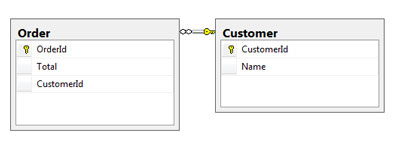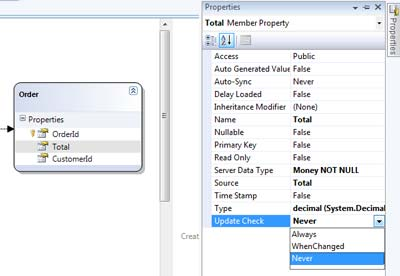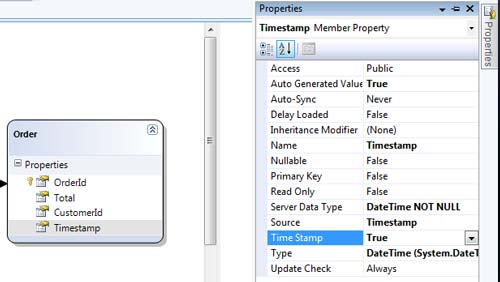# LINQ to SQL: How to convert Subsonic project to LINQ to SQL?

About a year and half I started to use Subsonic. This is a great tool. It made my life easier.? However, I kept asking myself: “Why Microsoft doesn’t have anything like this?”. Then I heard about LINQ. Finally, in February Visual Studio 2008 was released. However, still almost nobody uses LINQ to SQL in commercial projects. I did several attempts to convert some of my projects (one of those attempts is this website) from Subsonic to LINQ to SQL.

Let’s take a simple database:Let’s write a simple code for the order update.

Subsonic code would look like this:

``````public static Order GetOrder(int id)
{
return new Order(id);
}

public static Save(Order o)
{
o.Save(User.Identity.Name);
}
``````

and then you can do:

``````Order order = GetOrder(10);
order.Total = 12;
Save(order);
``````

Simple enough. Let’s try to do the same thing with LINQ to SQL. The key point is that load and update should be hidden in methods, so we can implement a three-tier structure of the project. So, let’s try to break the following code on separate methods:

``````DbDataContext db = newDbDataContext();
Order order = db.Orders.SingleOrDefault(o =&gt; o.OrderId == 10);
order.Total = 12;
db.SubmitChanges();
``````

Sounds simple, but unfortunately it’s really difficult. DataContext keeps track of all changes that happen to an object, so an object needs to be attached to DataContext in order to submit changes. The first idea is to create a global DataContext and use it for any kind of data manipulation. However, this is not simple at all. According to Microsoft’s best practices, you should use DataContext transitionally for small iterations. If you try to cheat, then you going to face a lot of hardly reproducible exceptions. Rick Strahl wrote a great article about this, Linq to SQL DataContext Lifetime Management. He created his own solution for global DataContext which he attached to a current thread. His solution works, but it is not trivial and definitely, and Microsoft didn’t intend this kind LINQ to SQL usage.

So, you need to use different DataContext for load and save operations. In order to do this you need to be able to detach and attach an object from its DataContext. Something like this:

``````private Order GetOrder(int id)
{
using (DbDataContext db = new DbDataContext())
{
Order o = db.Orders.SingleOrDefault(ord =&gt; ord.OrderId == id);
return o;
}
}

private void Save(Order o)
{
using (DbDataContext db = new DbDataContext())
{
db.Orders.Attach(o);
db.SubmitChanges();
}
}
``````

Execution of following code:

``````Order order = GetOrder(10);
order.Total = 12;
Save(order);
``````

Produces the following exception:

An attempt has been made to Attach or Add an entity that is not new, perhaps having been loaded from another DataContext. This is not supported.

There are two solutions to this problem:

1. Supply original object
``````private Order GetOrder(int id)
{
using (DbDataContext db = new DbDataContext())
{
Order o = db.Orders.SingleOrDefault(ord =&gt; ord.OrderId == id);
return o;
}
}

private void Save(Order o)
{
using (DbDataContext db = new DbDataContext())
{
Order oldOrder = GetOrder(o.OrderId);
db.Orders.Attach(o,oldOrder);
db.SubmitChanges();
}
}
``````

Where `db.DeferredLoadingEnabled = false` disables a relation between Order and Customer, otherwise, you going to get the following exception:

System.NotSupportedException: An attempt has been made to Attach or Add an entity that is not new, perhaps having been loaded from another DataContext. This is not supported.

P.S. If you don’t want to use `DeferredLoadingEnabled = false` then you can manually detach the object from a DataContext, like it’s described in Linq to SQL: How to Attach object to a different data context.. Ideally, LINQ to SQL should have a detach method like LINQ to Entities.

This creates a little bit of dirty SQL code:

``````UPDATE [dbo].[Order]
SET [Total] = @p3
WHERE ([OrderId] = @p0) AND ([Total] = @p1) AND ([CustomerId] = @p2)
-- @p0: Input Int (Size = 0; Prec = 0; Scale = 0) 
-- @p1: Input Money (Size = 0; Prec = 19; Scale = 4) [55.0000]
-- @p2: Input Int (Size = 0; Prec = 0; Scale = 0) 
-- @p3: Input Money (Size = 0; Prec = 19; Scale = 4) 
-- Context: SqlProvider(Sql2005) Model: AttributedMetaModel Build: 3.5.30729.1
``````

However, if you set Update Check to Never for each field in your tables then you are going to get the following SQL:``````UPDATE [dbo].[Order]
SET [Total] = @p1
WHERE [OrderId] = @p0
-- @p0: Input Int (Size = 0; Prec = 0; Scale = 0) 
-- @p1: Input Money (Size = 0; Prec = 19; Scale = 4) 
-- Context: SqlProvider(Sql2005) Model: AttributedMetaModel Build: 3.5.30729.1
``````

Not bad. Anyway Subsonic doesn’t do concurrency check. However, you are doing extra `select` in order to get an old object copy. Let’s see if we can avoid this.

1. Add timestamp field to each table``````private Order GetOrder(int id)
{
using (DbDataContext db = new DbDataContext())
{
Order o = db.Orders.SingleOrDefault(ord =&gt; ord.OrderId == id);
return o;
}
}

private void Save(Order o)
{
using (DbDataContext db = new DbDataContext())
{
db.Orders.Attach(o,true);
db.SubmitChanges();
}
}
``````

You still need to disable relations, but you don’t need to set Update Check to Never. LINQ to SQL does concurrency check based on a timestamp. As you see below we still have two SQL statements. However here select statement just gets a new timestamp value, not a whole object, which is more efficient.

``````UPDATE [dbo].[Order]
SET [Total] = @p2, [CustomerId] = @p3
WHERE ([OrderId] = @p0) AND ([Timestamp] = @p1)
SELECT [t1].[Timestamp]
FROM [dbo].[Order] AS [t1]
WHERE ((@@ROWCOUNT) &gt; 0) AND ([t1].[OrderId] = @p4)
-- @p0: Input Int (Size = 0; Prec = 0; Scale = 0) 
-- @p1: Input DateTime (Size = 0; Prec = 0; Scale = 0) [9/4/2008 9:14:31 PM]
-- @p2: Input Money (Size = 0; Prec = 19; Scale = 4) 
-- @p3: Input Int (Size = 0; Prec = 0; Scale = 0) 
-- @p4: Input Int (Size = 0; Prec = 0; Scale = 0) 
-- Context: SqlProvider(Sql2005) Model: AttributedMetaModel Build: 3.5.30729.1
``````

Conclusion:

As you can see you don’t need global DataContext to do simple CRUD operations. There are some tweaks that you need to do, but they are not too cruel.

Posted on May 2, 2008 by### Posted on 3/22/2009 06:48:44 PM by Ben Kloosterman

“As you can see you don’t need global DataContext to do simple CRUD operations. There are some tweaks that you need to do, but they are not too cruel.”

You do if you want good performance and its not that hard . Form apps can store a dataContext in the form ( or even a global) , web apps can store it in the session ( or thread) . Only for services does it get a bit harder here you really need to use the thread and you have to reapply changes made outside of the appdomain..I tried to use global DataContext. I’ve seen a really weird exceptions inside of DataContext class. My DataContext was attached to Application. If you attach DataContext to Session or Thread then you potentially going to face DataContext caching problems.

For example, one user made change to the article and then another user tried to change the same article. Both users would work with different DataContexts with cached versions of article.